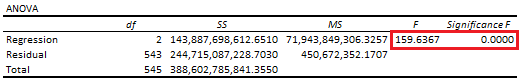# Linear Regression: Analysis of Variance ANOVA Table

Last Update: February 21, 2022

Linear Regression: Analysis of Variance ANOVA Table is used to analyze dependent variable  total variance together with its two components model fitted value  regression variance or explained variance and model random error  residual variance or unexplained variance. It is also used to evaluate whether adding independent variables improved linear regression model.

As example, we can fit a three-variable multiple linear regression with formula . Then, we can calculate ANOVA table regression degrees of freedom with formula , residual degrees of freedom with formula  and total degrees of freedom with formula  where is number of independent variables, is number of observations and is constant term. Next, we can estimate regression sum of squares with formula , residual sum of squares with formula and calculate total sum of squares with formula  where  are regression fitted values, is dependent variable mean, are dependent variable values and are regression residuals. After that, we estimate regression mean squared error with formula  and residual mean squared error with formula . Later, we estimate F-statistic with formula  and do F-test with joint null hypothesis that independent variables coefficients are equal to zero with formula . If joint null hypothesis is rejected, then adding independent variables and/or  improved linear regression model.

Below, we find an example of analysis of variance ANOVA table from multiple linear regression of house price explained by its lot size and number of bedrooms .Table 1. Microsoft Excel® analysis of variance ANOVA table from multiple linear regression of house price explained by its lot size and number of bedrooms.

Courses

My online courses are hosted at Teachable website.

For more details on this concept, you can view my Linear Regression Courses.

 Data Description: Sales prices of houses sold in the city of Windsor, Canada, during July, August and September, 1987.

Original Source: Anglin, P., and Gencay, R. (1996). Semiparametric Estimation of a Hedonic Price Function. Journal of Applied Econometrics, 11, 633–648.

Source: AER R Package HousePrices Object. Christian Kleiber and Achim Zeileis. (2008). Applied Econometrics with R. Springer-Verlag, New York.

+